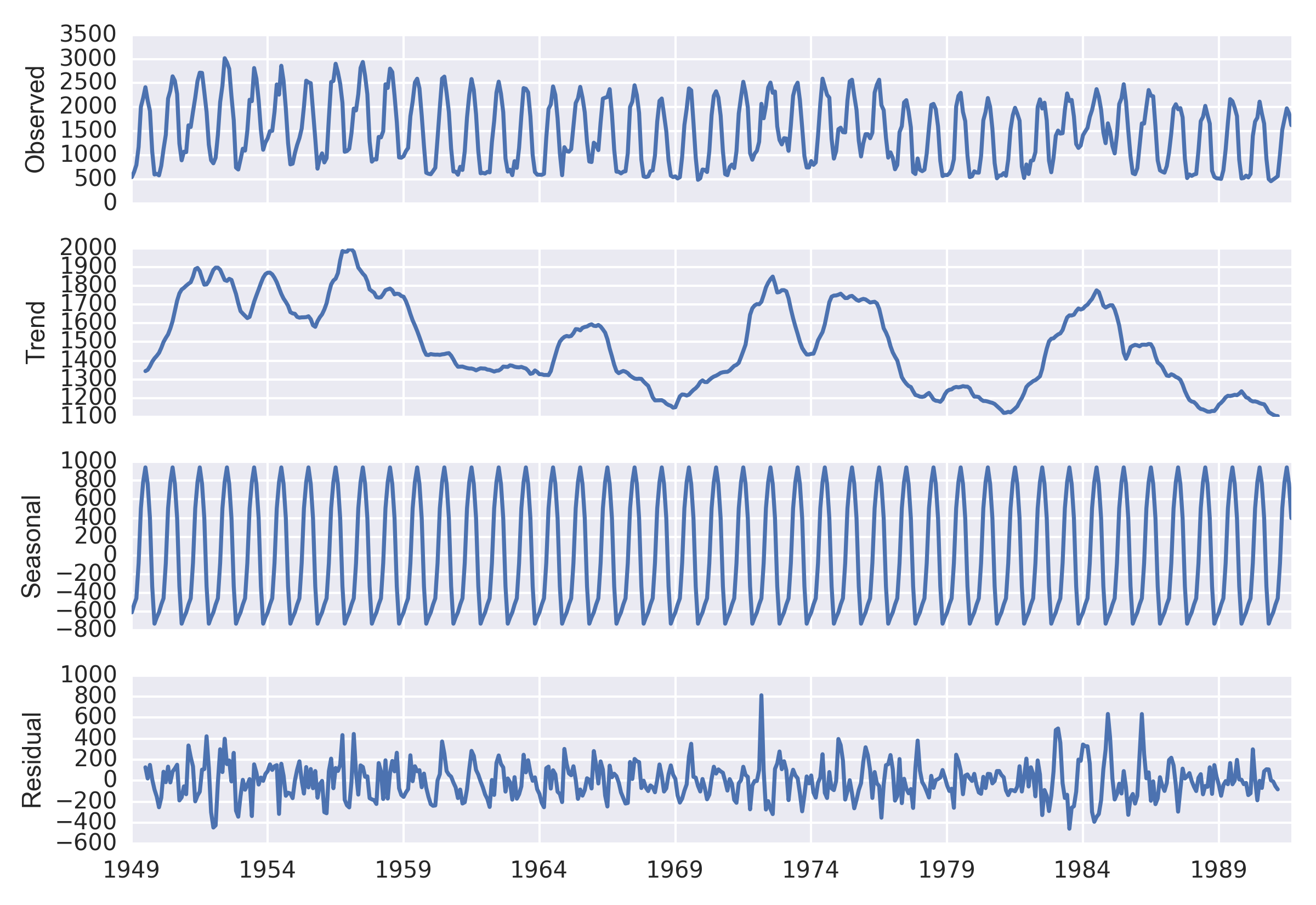# Time-series decomposition and trend analysis in Python

Decompose time series in Python and a function for the Mann-Kendall test for trend.

Michael Schramm https://michaelpaulschramm.com (Texas Water Resources Institute)https://twri.tamu.edu
August 1, 2015

There are a number of methods to accomplish time-series decompositions in R, including the `decompose` and `STL` commands.

I haven’t come across a seasonal decomposition method in Python comparable to R’s `STL`. However, statsmodels 0.6 added a naive seasonal decomposition method similar to R’s `decompose` that is not as powerful as the LOESS method used in `STL`. Let’s run through an example:

``````
import urllib2
import datetime as datetime
import pandas as pd
import statsmodels.api as sm
import seaborn as sns
import matplotlib.pyplot as plt

# Import the sample streamflow dataset
data = urllib2.urlopen('https://raw.github.com/mps9506/Sample-Datasets/master/Streamflow/USGS-Monthly_Streamflow_Bend_OR.tsv')
df = pd.read_csv(data, sep='\t')

# The yyyy,mm, and dd are in seperate columns, we need to make this a single column
df['dti'] = df[['year_nu','month_nu','dd_nu']].apply(lambda x: datetime.datetime(*x),axis=1)

# Let use this as our index since we are using pandas
df.index = pd.DatetimeIndex(df['dti'])
# Clean the dataframe a bit
df = df.drop(['dd_nu','year_nu','month_nu','dti'],axis=1)
df = df.resample('M',how='mean')
print df.head()
fig,ax = plt.subplots(1,1, figsize=(6,4))
flow = df['mean_va']
flow = flow['1949-01':]

res = sm.tsa.seasonal_decompose(flow)
fig = res.plot()
fig.show()  ``````decompose

Each component can then be accessed with:

``````
residual = res.residual
seasonal = res.seasonal
trend = res.trend
print trend['1950':'1951']``````
``````
1950-01-31    1441.591667
1950-02-28    1468.133333
1950-03-31    1499.883333
1950-04-30    1521.466667
1950-05-31    1540.633333
1950-06-30    1572.079167
1950-07-31    1611.412500
1950-08-31    1666.541667
1950-09-30    1720.658333
1950-10-31    1759.700000
1950-11-30    1780.408333
1950-12-31    1789.491667
1951-01-31    1800.950000
1951-02-28    1810.950000
1951-03-31    1819.616667
1951-04-30    1848.866667
1951-05-31    1889.850000
1951-06-30    1895.979167
1951-07-31    1878.858333
1951-08-31    1841.137500
1951-09-30    1806.308333
1951-10-31    1807.850000
1951-11-30    1826.516667
1951-12-31    1856.683333
Freq: M, Name: mean_va, dtype: float64  ``````

If we want to determine if there is a simple monotonic trend in this data we can utilize the Mann-Kendall test for trend. This doesn’t appear to be available in scipy.stats or statsmodels yet. I came across a function written by Sat Kumar Tomer, the homepage with the software package seems to be gone, so I verified the output to implementations in R and uploaded to GitHub so it won’t disappear.

``````
import numpy as np
from scipy.stats import norm, mstats

def mk_test(x, alpha = 0.05):
"""
Input:
x:   a vector of data
alpha: significance level (0.05 default)

Output:
trend: tells the trend (increasing, decreasing or no trend)
h: True (if trend is present) or False (if trend is absence)
p: p value of the significance test
z: normalized test statistics

Examples
--------
>>> x = np.random.rand(100)
>>> trend,h,p,z = mk_test(x,0.05)
"""
n = len(x)

# calculate S
s = 0
for k in range(n-1):
for j in range(k+1,n):
s += np.sign(x[j] - x[k])

# calculate the unique data
unique_x = np.unique(x)
g = len(unique_x)

# calculate the var(s)
if n == g: # there is no tie
var_s = (n*(n-1)*(2*n+5))/18
else: # there are some ties in data
tp = np.zeros(unique_x.shape)
for i in range(len(unique_x)):
tp[i] = sum(unique_x[i] == x)
var_s = (n*(n-1)*(2*n+5) + np.sum(tp*(tp-1)*(2*tp+5)))/18

if s>0:
z = (s - 1)/np.sqrt(var_s)
elif s == 0:
z = 0
elif s<0:
z = (s + 1)/np.sqrt(var_s)

# calculate the p_value
p = 2*(1-norm.cdf(abs(z))) # two tail test
h = abs(z) > norm.ppf(1-alpha/2)

if (z<0) and h:
trend = 'decreasing'
elif (z>0) and h:
trend = 'increasing'
else:
trend = 'no trend'

return trend, h, p, z``````

Let’s see if there is a trend direction in the first decade of data 1950-1960:

``````
trend = res.trend['1950':'1960']
test_trend,h,p,z = mk_test(trend,alpha=0.05)
print test_trend, h
print z, p  ``````
``````
decreasing True
-4.05429896945 5.02848722452e-05``````

The test indicates a monotonic decreasing trend over the time period, with a Mann-Kendall Z stat = -4.05 and p<0.05.

### Corrections

If you see mistakes or want to suggest changes, please create an issue on the source repository.

### Reuse

Text and figures are licensed under Creative Commons Attribution CC BY 4.0. Source code is available at https://github.com/mps9506/mschramm, unless otherwise noted. The figures that have been reused from other sources don't fall under this license and can be recognized by a note in their caption: "Figure from ...".

### Citation

For attribution, please cite this work as

`Schramm (2015, Aug. 1). @mpschramm: Time-series decomposition and trend analysis in Python. Retrieved from https://michaelpaulschramm.com/posts/time-series-python/`

BibTeX citation

```@misc{schramm2015time-series,
author = {Schramm, Michael},
title = {@mpschramm: Time-series decomposition and trend analysis in Python},
url = {https://michaelpaulschramm.com/posts/time-series-python/},
year = {2015}
}```